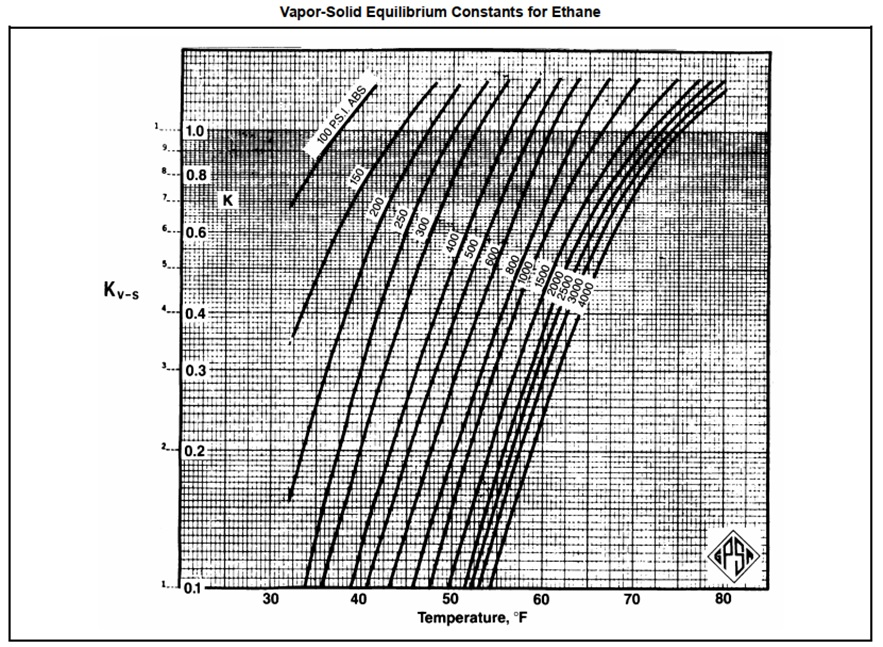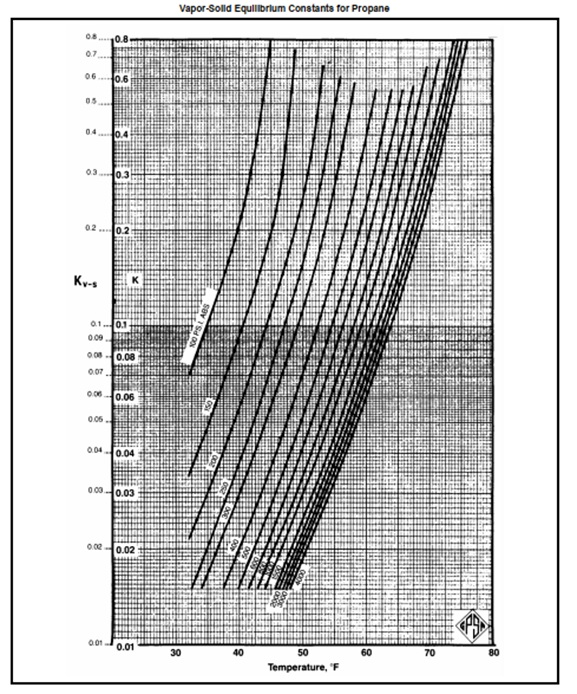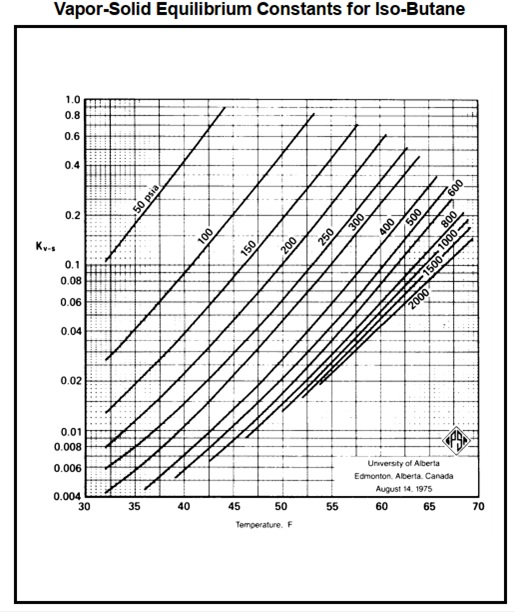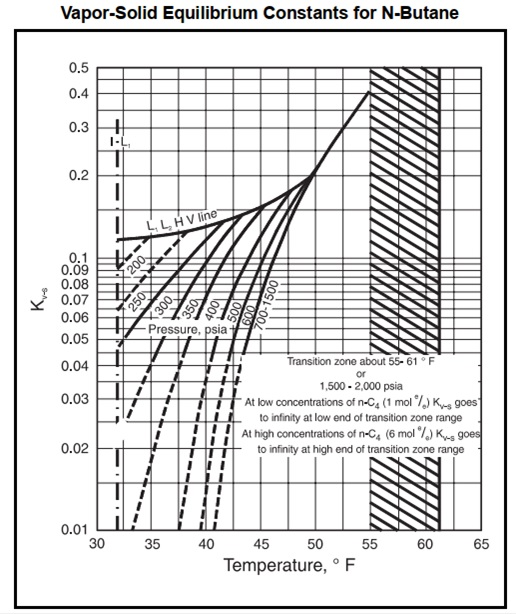# Hydrate Prediction using Vapor-Solid Equilibrium Constant

In previous post, hydrate in natural gas system can be estimated by two approaches, which are approximate method and analytical method. Approximate method used when gas composition is unknown. It uses pressure-temperature correlation to predict hydrate formation temperature.

In this post, I want to share how to predict hydrate formation using analytical method, which uses vapor-solid equilibrium constant. Vapor-solid equilibrium constant is expressed as below:Where

Ki = equilibrium constant for component i

Yi = mol fraction of each component in the gas on a water free basis

Xi = mol fraction of each component in the solid on a water free basis

K-value for each hydrate forming molecule (methane, ethane, propane, isobutane, normal butane, carbon dioxide, and hydrogen sulfide) are shown below. Normal butane cannot form a hydrate by itself but can contribute to hydrate formation in a mixture.To make calculation as simple as possible, all molecules too large to form hydrates have a K-value of infinity. These include all normal paraffin hydrocarbon larger than normal butane. Nitrogen is assumed to be non-hydrate former and is also assigned a K-value of infinity.

Hydrate prediction using vapor-solid constant is iterative. Convergence is achieved when the following condition is met.Flow chart below shows how to estimate hydrate formation temperature using vapor-solid correlation.Example

Let say a natural gas has composition below:Operating temperature is 50oF. What is hydrate formation pressure?

Solution:

Before predicting hydrate formation pressure using vapor-solid equilibrium, let’s estimate hydrate formation pressure using approximate method. We found that based on composition above, gas specific gravity is 0.7. At 50oF, hydrate formation pressure is 320 psia.Then, we estimate hydrate formation pressure using vapor-solid constants. We choose pressure 300 psia and 400 psia because we got hydrate formation pressure is approximately 320 psia by using approximate method (pressure-temperature correlation).

At 300 psia and 400 psia we got Yi/Ki below.To get total Yi/Ki = 1, we interpolate the value, then we get hydrate formation pressure is 309 psia.

Spreadsheet below shows example on how to predict hydrate formation based on steps above.

Hydrate prediction vapor-solid constant

I hope you find this post useful.

References:

Stewart, M., & Arnold, K. (2011). Gas Dehydration Field Manual. Gulf Professional Publishing.

GPSA Engineering Data Book